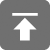# 工学1号馆

home

## 直接插入排序和折半插入排序算法

Wu Yudong    July 25, 2016     Algorithm   539

1. 初始时，a自成1个有序区，无序区为a[1..n-1]。令i=1

2. 将a[i]并入当前的有序区a[0…i-1]中形成a[0…i]的有序区间。

3. i++并重复第二步直到i==n-1。排序完成。void InsertSort(int *a, int n)
{
int i,j,k;
for(i = 1; i < n; i++) {
for(j = i - 1; j >= 0; j--){
if(a[j] < a[i]) break;
}

if(j != i - 1) {
int temp = a[i];
for(k = i - 1; k >j; k--)
a[k + 1] = a[k];
a[k + 1] = temp;
}
}
}

void BinaryInsertSort(int *a, int n)
{
int i, j, k, low, high, m;
for(i = 1; i < n; i++) {
low = 0;
high = i - 1;

while(low <= high) {
m = (low + high) / 2;
if(a[m] > a[i]) high = m - 1;
else low = m + 1;
}

if(j != i - 1) {
int temp = a[i];
for(k = i - 1; k >= high + 1; k--)
a[k + 1] = a[k];
a[k + 1] = temp;
}
}
}